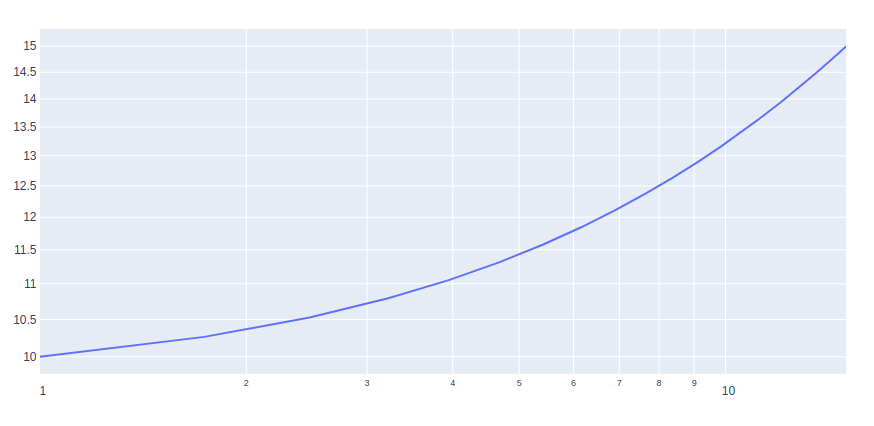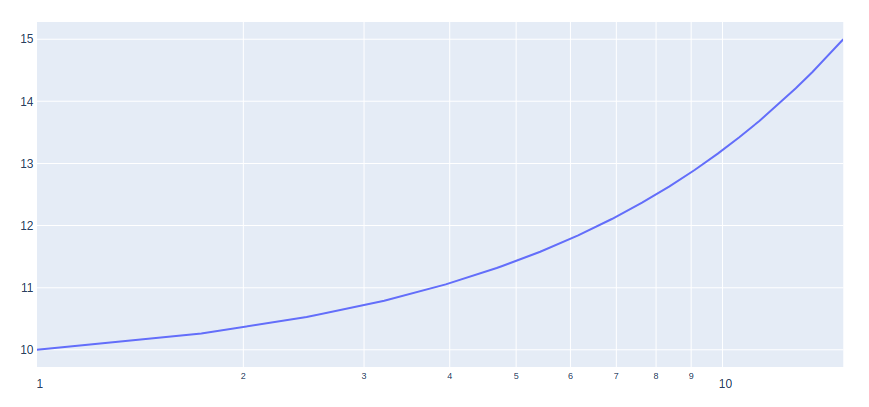# How to make Log Plots in Plotly – Python?

A Plotly is a Python library that is used to design graphs, especially interactive graphs. It can plot various graphs and charts like histogram, barplot, boxplot, spreadplot, and many more. It is mainly used in data analysis as well as financial analysis. plotly is an interactive visualization library.

## What are the log plots?

A log plot is a way of displaying numerical data over a very wide range of values in a compact way. There are two types of plots:

• log-log plot: If both the vertical and horizontal axes of a plot are scaled logarithmically, the plot is referred to as a log-log plot.
• Semi-logarithmic plots: If only the ordinate or abscissa is scaled logarithmically, the plot is referred to as a semi-logarithmic plot.

### Creating Log plots using plotly

There is no specific function provided for creating the log plots. However, it can be created using the scatter() method of graph_objects class. We will have to change the xaxis_type and yaxis_type to log.

Example 1: log-log plot

## Python3

 `import` `plotly.graph_objects as go ` `import` `numpy as np ` ` `  ` `  `x ``=` `np.linspace(``1``, ``15``, ``20``) ` `y ``=` `np.linspace(``10``, ``15``, ``20``) ` ` `  `fig ``=` `go.Figure() ` ` `  `fig.add_trace(go.Scatter( ` `    ``x``=``x, ` `    ``y``=``y ` `)) ` ` `  `fig.update_layout(xaxis_type``=``"log"``, yaxis_type``=``"log"``) ` `fig.show() `

Output:Example 2: semi-log plot

## Python3

 `import` `plotly.graph_objects as go ` `import` `numpy as np ` ` `  ` `  `x ``=` `np.linspace(``1``, ``15``, ``20``) ` `y ``=` `np.linspace(``10``, ``15``, ``20``) ` ` `  `fig ``=` `go.Figure() ` ` `  `fig.add_trace(go.Scatter( ` `    ``x``=``x, ` `    ``y``=``y ` `)) ` ` `  `fig.update_layout(xaxis_type``=``"log"``) ` `fig.show()`

Output:Attention geek! Strengthen your foundations with the Python Programming Foundation Course and learn the basics.

To begin with, your interview preparations Enhance your Data Structures concepts with the Python DS Course.

My Personal Notes arrow_drop_upIf you like GeeksforGeeks and would like to contribute, you can also write an article using contribute.geeksforgeeks.org or mail your article to contribute@geeksforgeeks.org. See your article appearing on the GeeksforGeeks main page and help other Geeks.

Please Improve this article if you find anything incorrect by clicking on the "Improve Article" button below.

Article Tags :

Be the First to upvote.

Please write to us at contribute@geeksforgeeks.org to report any issue with the above content.